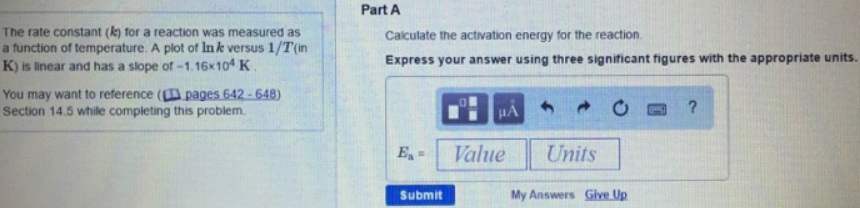# Problem: The rate constant (k) for a reaction was measured as a function of temperature. A plot of ln k versus 1/T (in K) is linear and has a slope of -1.16 x 104 K. Calculate the activation energy for the reaction. Express your answer using three significant figures with the appropriate units.

###### FREE Expert Solution
79% (80 ratings)###### Problem Details

The rate constant (k) for a reaction was measured as a function of temperature. A plot of ln k versus 1/T (in K) is linear and has a slope of -1.16 x 104 K.

Calculate the activation energy for the reaction.